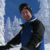# Why Are Linear Plots Mostly Used in Regression?

Six Sigma – iSixSigma Forums General Forums Tools & Templates Why Are Linear Plots Mostly Used in Regression?

Viewing 4 posts - 1 through 4 (of 4 total)
• Author
Posts
• #239874vivek_6m
Participant

why linear plots are mostly used in regression than quadratic and cubes

0
#239875Robert Butler
Participant

I’m not trying to be sarcastic but who is making the claim that linear plots are mostly used in [an examination?] of a linear regression?

There are any number of reasons why an analysis might result in employing simple linear plots. For example:
1. Your plan for analysis involves running a two level factorial design. – In this case, by definition, a simple linear plot is all you are going to get.
2. A plot of your production data says the the trend is linear – therefore if you run a regression on the data linear is going to be the best fit.
3. Even though your knowledge of the process says the relationship between your independent and dependent variables is some sort of curvilinear process, the region where you happen to be running your process is a region where the relationship between the independent and dependent variables are essentially linear.

If you are going to look at curvilinear trending then, at the very minimum, you need to have 3 levels for your independent variables. One of the reasons you run center points on two-level factorial designs (we are assuming the X’s are or can be treated as continuous) is to check for possible deviations from linear. If you find this to be the case then one will often augment the existing design to check for some kind of curvilinear response (usually quadratic/logarithmic)and your final plots will be curvilinear in nature.

As for cubic (or higher) – the first question you need to ask is when would you expect to encounter such behavior? If you are actually working on something and plots of your production data do suggest you have a real cubic response then you would want to build a design with 4 levels for the various continuous independent variables.

In all of the years of process analysis the only time I ever encountered a genuine cubic response was when we found out the region we were examining resulted in a phase change of the output (we were adding a rubberized compound to a plastic mix and we crossed the 50% threshold which meant we went from rubberized plastic to plasticized rubber). In short, in my experience, real cubic and higher curvilinear responses are extremely rare.

There is, of course, data that is cyclic in nature such as monthly changes in demand for a product and I suppose you could try to model data of this type using linear regression methods but the first line of defense when attacking this kind of data is time series analysis and a discussion of that tool is, I think, beyond the focus of your question.

0
#240156Rhb
Participant

I generally use Quadratic Regression because there’s almost always some curvature in data distributions. Just my preference, but I typically find it to be the best fit.

0
#240160Chuck White
Participant

I have to disagree with the idea of using a quadratic model by default. Adding terms to a regression model will always increase the R-square, and therefore make the model fit the data better. But a higher R-square doesn’t mean the predictive power of the model is any better. In fact, any terms that are just fitting the noise of the data set can actually make the predictive power worse. You should always check the significance of the terms, and also compare adjusted and predictive R-squared values if available.

In the words of George Box, all models are wrong, but some are useful. In my experience, the simplest model with adequate predictive power tends to be the most useful.

0
Viewing 4 posts - 1 through 4 (of 4 total)

You must be logged in to reply to this topic.